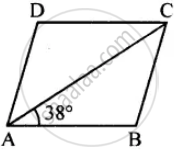# Abcd is a Rhombus. If ∠Bac = 38°, Find : (I) ∠Acb (Ii) ∠Dac (Iii) ∠Adc. - Mathematics

Sum

ABCD is a rhombus. If ∠BAC = 38°, find :
(i) ∠ACB
(ii) ∠DAC#### Solution

ABCD is Rhombus (Given)

AB = BC

∠BAC = ∠ACB (∠s opp. to equal sides)

But ∠BAC = 38° (Given)

∠ACB = 38°

In ∆ABC,

∠ABC + ∠BAC + ∠ACB = 180°

∠ABC + 38°+ 38° = 180°

∠ABC = 180° – 76° = 104°

But ∠ABC = ∠ADC (opp. ∠s of rhombus)

∠DAC = ∠DCA ( AD = CD)

∠DAC = 1/2 [180° - 104°]

∠DAC = 1/2 xx 76° = 38°

Hence (i) ∠ACB = 38° (ii) ∠DAC = 38° (iii) ∠ADC = 104° Ans.

Concept: Types of Quadrilaterals - Properties of Rhombus
Is there an error in this question or solution?

#### APPEARS IN

Selina Concise Mathematics Class 8 ICSE
Chapter 17 Special Types of Quadrilaterals
Exercise 17 | Q 6 | Page 198